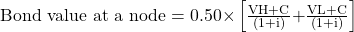Essential Concept 65: Pricing a Bond using a Binomial Tree | IFT World
101 Concepts for the Level I Exam

# Essential Concept 65: Pricing a Bond using a Binomial TreeTo find the value of the bond at a particular node, we use the backward induction valuation methodology.

Backward Induction: Start at maturity, fill in those values, and work back from right to left to find the bond’s value at the desired node. Refer to the following figure.

The value of the bond is given by the following equation: